Author: Oscar Cronquist Article last updated on July 05, 2022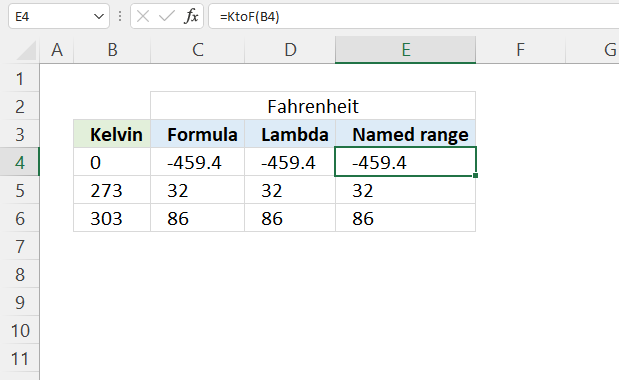The LAMBDA function lets you build custom functions using only regular Excel functions, no VBA is needed. These custom functions based on the LAMBDA function are available only in your workbook.

The Name Manager lets you create a unique name for your custom LAMBDA function. What makes the LAMBDA function really powerful is its ability to create recursive formulas.

## 1. LAMBDA Function Syntax

LAMBDA([parameter1, parameter2, …,] calculation)

## 2. LAMBDA Function Arguments

 parameter1 Optional. A value that you want to use in the custom function, you are allowed to use a cell reference, string, or number. Up to 253 parameters. calculation Required. A formula to evaluate. Must be the last argument, a result is also required.

## 3. LAMBDA Function ExampleThis example demonstrates how to build a custom LAMBDA function, there are three main steps.

1. Create a formula.
2. Build the LAMBDA function.
3. Name the LAMBDA function.

We are going to build a custom LAMBDA function that converts Kelvin to Fahrenheit, it is a simple calculation and works great as a demonstration.

### 3.1 Create a formula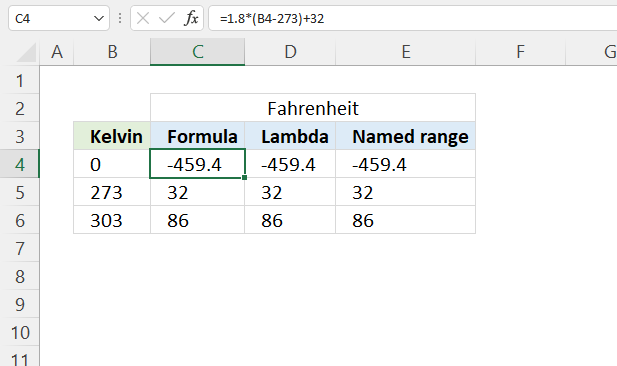This example shows how to convert Kelvin to Fahrenheit, the calculation is: F = 1.8(K - 273) + 32

Formula in cell C4:

=1.8*(B4-273)+32

### Explaining formula

#### Step 1 - Subtract Kelvin with 273

The minus operator lets you subtract numbers in an Excel formula.

B4-273

0-273 equals -273

#### Step 2 - Multiply by 1.8

The asterisk character lets you multiply numbers in an Excel formula. The parentheses allow you to control the order of operation.

1.8*(B4-273)

becomes

1.8*-273 equals -491.4

#### Step 3 - Add 32

The plus operator lets you add numbers in an Excel formula.

1.8*(B4-273)+32

becomes

-491.4 + 32 equals -459.4

### 3.2 Build the LAMBDA function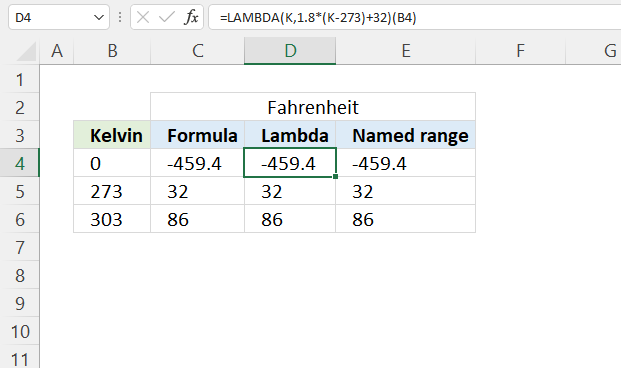Formula in cell D4:

=LAMBDA(K,1.8*(K-273)+32)(B4)

### Explaining formula

#### Step 1 - Formula

This is the formula we built in section 3.1 There is only one input value in this formula.

1.8*(B4-273)+32

#### Step 2 - LAMBDA function

The LAMBDA function has up to 253 parameters, however, we need only one parameter, in this example named K.

LAMBDA([parameter1, parameter2, …,] calculation)

LAMBDA(K, 1.8*(K-273)+32)

The bolded part above is the formula we built in section 3.1, cell reference B4 is replaced with parameter K.

#### Step 3 - Pass a value to the LAMBDA function

The LAMBDA function uses parentheses to pass values to the LAMBDA formula.

LAMBDA(K,1.8*(K-273)+32)(B4)

The parameters must be in the same order as you specified them, in this case, it doesn't matter. There is only one parameter.

### 3.3 Name the LAMBDA functionExcel feature "Named ranges" allows us to assign a custom name to the LAMBDA function. I named it KtoF, shown in cell E4 in the image above.

Formula in cell E4:

=KtoF(B4)

### How to assign a name to a LAMBDA function

1. Go to tab "Formulas" on the ribbon.
2. Press with left mouse button on "Name Manager"  button. A dialog box appears.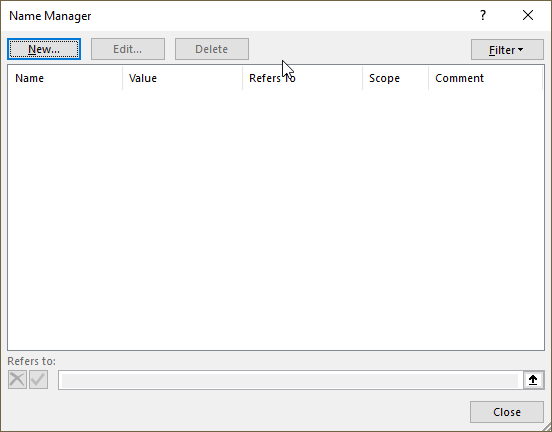3. Press with left mouse button on "New.." button. Another dialog box appears.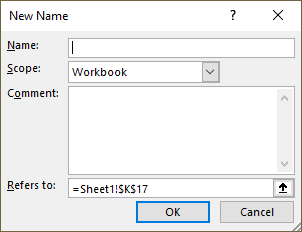4. Type a name.
5. Paste the LAMBDA function to "Refers to:" field.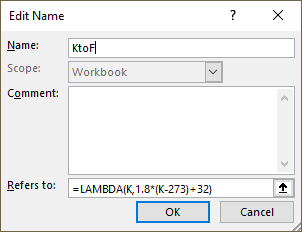6. Press with left mouse button on the "OK" button.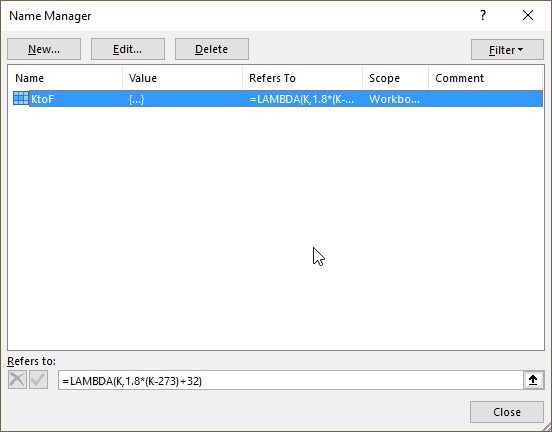7. Press with left mouse button on the "Close" button.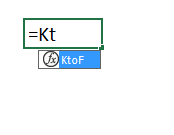The function we created appears if we type the first characters in the function name in a cell.